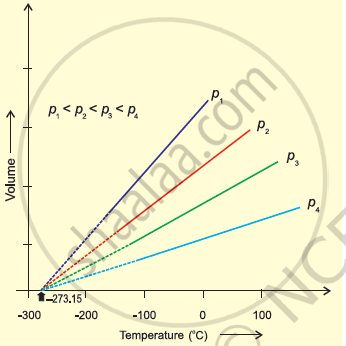Share

# In Terms of Charles’ Law Explain Why –273°C is the Lowest Possible Temperature. - Chemistry

#### Question

In terms of Charles’ law explain why –273°C is the lowest possible temperature.

#### Solution 1

Charles’ law states that at constant pressure, the volume of a fixed mass of gas is directly proportional to its absolute temperature.It was found that for all gases (at any given pressure), the plots of volume vs. temperature (in °C) is a straight line. If this line is extended to zero volume, then it intersects the temperature-axis at – 273°C. In other words, the volume of any gas at –273°C is zero. This is because all gases get liquefied before reaching a temperature of – 273°C. Hence, it can be concluded that – 273°C is the lowest possible temperature.

#### Solution 2

At -273°C, volume of the gas becomes equal to zero, i.e., the gas ceases to exist.

Is there an error in this question or solution?